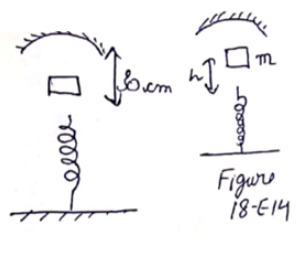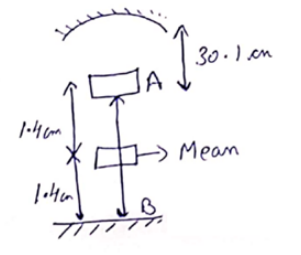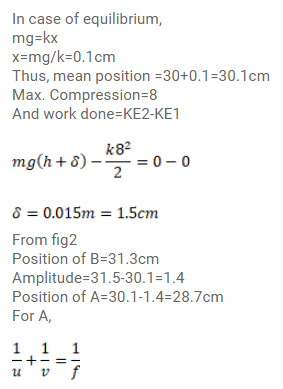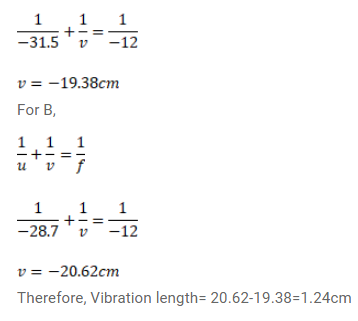# A mass m=50g is dropped on a vertical spring of spring constant

Question:

A mass $m=50 \mathrm{~g}$ is dropped on a vertical spring of spring constant $500 \mathrm{~N} / \mathrm{m}$ from a height $\mathrm{h}=10 \mathrm{~cm}$ as shown in fig. The mass sticks to the spring ans executes simple harmonic oscillation after that. A concave mirror of focal length $12 \mathrm{~cm}$ facing the mass is fixed with its principal axis coinciding with the line of motion of the mass, its pole being at a distance of $30 \mathrm{~cm}$ from the free end of the spring. Find the length in which the image of the mass oscillates.Solution: